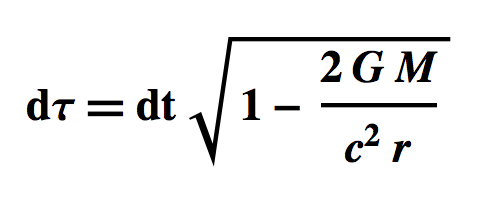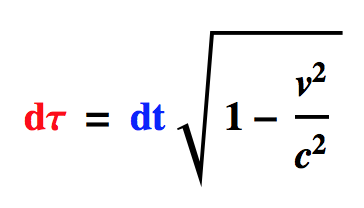# GPS and Time Dilation

The GPS system incorporates both Special and General Relativity. Otherwise it wouldn’t work.

#### Global Positioning System

• The Global Positioning System (GPS) is a space-based radio-navigation system that broadcasts highly accurate navigation pulses to users on Earth. The system consists of 24 satellites orbiting at 20,200 km (12,550 miles). Each satellite circles the Earth twice daily.
• The satellites in the GPS constellation are arranged in six equally-spaced orbital planes surrounding the Earth. Four satellites occupy each plane.
• This arrangement ensures GPS receivers can view at least four satellites from virtually any point on the planet.

#### Trilateration

• If you know the distance to three orbiting satellites and their locations, you can calculate your position on Earth’s surface by Trilateration.
• Brown Earth with a Red Satellite
• The radius of the Red Sphere is the distance between the Red Satellite and a GPS receiver on the Earth’s Surface (calculated by the GPS receiver).
• So the GPS receiver is located anywhere on the circle formed by the intersection of the Earth and the Red Sphere.
• Brown Earth with Red and Blue Satellites
• Using the Red Satellite the GPS receiver determines that its location is on the circle formed by the intersection of the Earth and the Red Sphere.
• The Blue Sphere intersects the Earth-Red circle at two points, narrowing the location of the GPS receiver to two points on the Earth’s surface.
• A third satellite fixes the location at one of those points.
• So a GPS receiver must determine the location and distance to at least three satellites.
• Location
• A GPS satellite transmits its location to GPS receivers.
• Distance
• A receiver computes the distance (d = vt) to a satellite by multiplying
• the time it takes a signal to reach the receiver from the satellite (less than a tenth of a second.)
• AND
• The velocity of light
• In the GPS system signals go only one way, from satellite to receiver.  So a receiver does not determine elapsed time by bouncing a signal off the satellite.
• How Stuff Works: GPS Receivers
• At a particular time (let’s say midnight), the satellite begins transmitting a long, digital pattern called a pseudo-random code. The receiver begins running the same digital pattern also at midnight. When the satellite’s signal reaches the receiver, its transmission of the pattern will lag a bit behind the receiver’s playing of the pattern.
• [Lamb: Imagine that the satellite clock and receiver clock start counting simultaneously at midnight: “1 Mississippi, 2 Mississippi, 3 Mississippi …”  If the satellite transmits the words “7 Mississippi” and the receiver receives the message when it’s on “8 Mississippi,” the elapsed time is “1 Mississippi.”]
• To accurately measure the elapsed time, the receiver and satellite both need clocks that can be synchronized to the nanosecond. To make a satellite positioning system using only synchronized clocks, you would need to have atomic clocks not only on the satellites, but also in the receiver itself. But atomic clocks cost between \$50,000 and \$100,000, making them a bit too expensive for everyday consumer use.
• The Global Positioning System has a clever, effective solution to this problem. Every satellite contains an expensive atomic clock, but the receiver uses an ordinary quartz clock, which it constantly resets. The receiver thus looks at incoming signals from four or more satellites to compensate for its imperfect timekeeping.

#### Two Kinds of Time Dilation

• Because of Time Dilation the atomic clocks on the GPS satellites run faster than those on the ground by enough that the GPS system must account for the difference.
• Two Kinds of Time Dilation
• Gravitational Time Dilation (General Relativity)
• GR predicts that time passes more slowly in a gravitational field
• GR predicts GPS satellites gain 45 microseconds a day.
• Kinetic Time Dilation (Special Relativity)
• SR predicts that time passes more slowly on moving objects
• SR predicts GPS satellites lose 7 microseconds a day.
• So GR and SR together predict GPS satellites gain 38 microseconds a day.
• (The best atomic clocks are predicted to be off by less than one second in 50 million years.)

#### General Relativity Calculation of +45 ms

• Assume a universe consisting of just the Earth, three atomic clocks, and your spaceship.  The first clock is on the surface of the Earth; the second is hovering 20,000 km above; and the third is on your spaceship, far away from Earth’s gravity.
• You define a reference frame using spherical coordinates (ct,r,θ,ϕ), with the origin at the center of Earth and coordinate time t kept by your atomic clock.
• Assume the surface clock is at (r,θ,ϕ), where r = the radius of the earth, θ = 0, and ϕ = 0.
• Assume the  up-in-the-air clock is at (r,θ,ϕ), where r = the radius of the earth + 20,000 km, θ = 0, and ϕ = 0.
• The Schwarzschild metric can be used to calculate how many microseconds the surface clock loses in 24 hours compared to the up-in-the-air clock
• Since  θ = 0, and ϕ = 0, it follows from the Schwarzschild line element that:• Where
• M is the mass of Earth
• G is the gravitational constant
• c is the speed of light
• dt is 24 hours per your atomic clock, i.e. 86400 seconds
• Suppose r is the distance from the center of Earth to the surface clock, 6,371,010 meters
• Then the elapsed time on the surface clock is:
• = 86399.9999398605240121871294527 seconds
• Suppose r is the distance from the center of Earth to the Up-in-the-air Clock, 6,371,010 + 20,000,000 meters
• Then the elapsed time on the Up-in-the-air Clock is:
• = 86399.9999854708180379900491482 seconds
• The Up-in-the-air Clock thus gains = 45.61 microseconds

#### Special Relativity Calculation of -7 ms

• The velocity of a GPS satellite is 14,000 km/hr relative to the Earth
• Which is, v = 3888.88 meters per second
• An atomic clock on Earth ticks off 86,400 seconds a day
• dt = 86400 seconds
• The speed of light is 299,792,458 meters per second
• c = 299,792,458
• The formula for the proper time in Special Relativity (derived from the Lorentz Transformation) is:• So, = 86399.9999927306863001438889650 seconds
• Thus, the GPS atomic clocks lose 7 microseconds a day relative to Earth’s atomic clocks
• (dt) x 1,000,000 = -7.269313699856111035 microseconds per day

#### Calculations Combined

• GR predicts that GPS satellites gain 45 microseconds daily due to Gravitational Time Dilation (time passing more slowly in a gravitational field)
• = +45.61 microseconds per day
• SR predicts GPS satellites lose 7 microseconds a day due to Kinetic Time Dilation (time passing more slowly in a moving reference frame).
• dt = -7.27 microseconds per day
• So GR and SR together predict GPS satellites gain 38 microseconds a day.